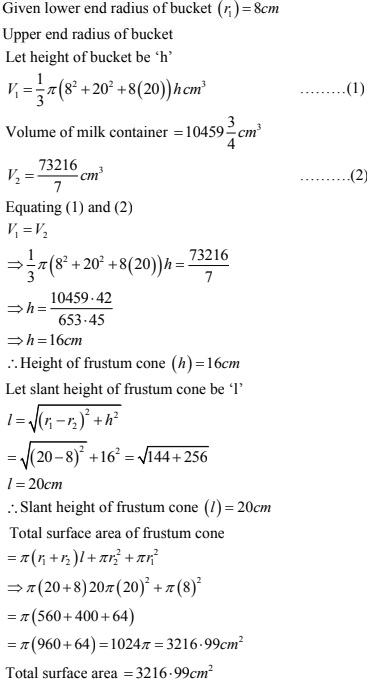# Chapter 16 Surface Areas and Volumes RD Sharma Solutions Exercise 16.3 Class 10 Maths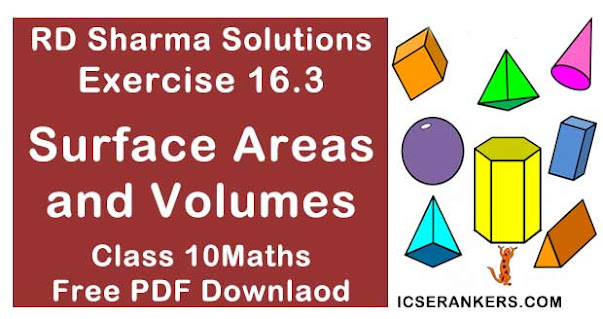Chapter Name RD Sharma Chapter 16 Surface Area and Volumes Exercise 16.1 Book Name RD Sharma Mathematics for Class 10 Other Exercises Exercise 16.1Exercise 16.2 Related Study NCERT Solutions for Class 10 Maths

### Exercise 16.3 Solutions

1. A bucket has top and bottom diameters of 40cm and 20 cm respectively. Find the volume of the bucket if its depth is 12 cm. Also, find the cost of tin sheet used for making the bucket at the rate of Rs 1.20 per dm2 . (Use π = 3.14)
Solution
Given diameter to top of bucket = 40cm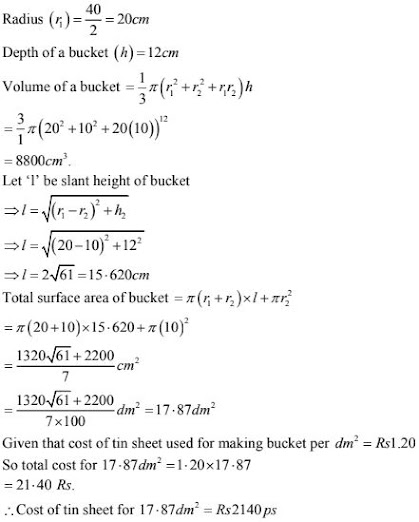2. A frustum of a right circular cone has a diameter of base 20 cm, of top 12cm and height 3cm. Find the area of its whole surface and volume ?
Solution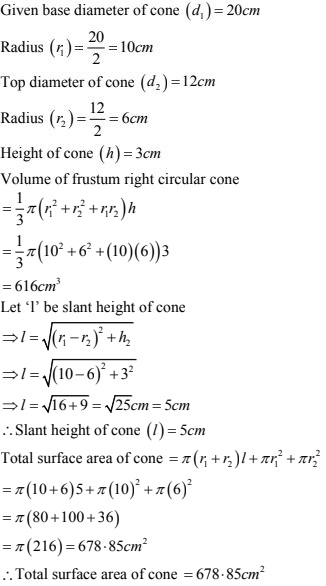3. The slant height of the frustum of a cone is 4cm and perimeters of it circular ends are 18cm and 6cm. Find curved surface of the frustum ?
Solution
Given slant height of cone (r) = 4 cm
Let radii of top and bottom circles be r1 and r2 .
Given perimeters of its ends as 18cm and 6cm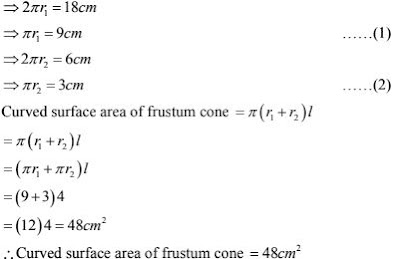4. The perimeters of the ends of a frustum of a right circular cone are 44 cm and 33cm. If the height of the frustum be 16 cm, find its volume, the slant surface and the total surface.
Solution
Given perimeters of ends of frustum right circular cone are 44cm and 33cm
Height of frustum cone = 16 cm
Perimeter = 2πr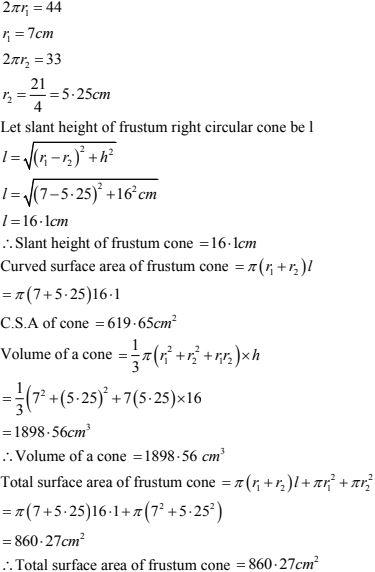5. If the radii of circular ends of a conical bucket which is 45cm high be 28cm and 7cm. Find the capacity of the bucket ?
Solution
Given height of conical bucket = 45cm
Give radii of 2 circular ends of a conical bucket is 28cm and 7cm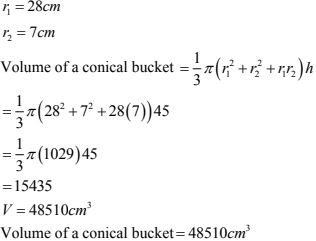6. The height of a cone is 20 cm. A small cone is cut off from the top by a plane parallel to the base. If its volumes be 1/25 of the volume of the original cone, determine at what height above base the section is made.
Solution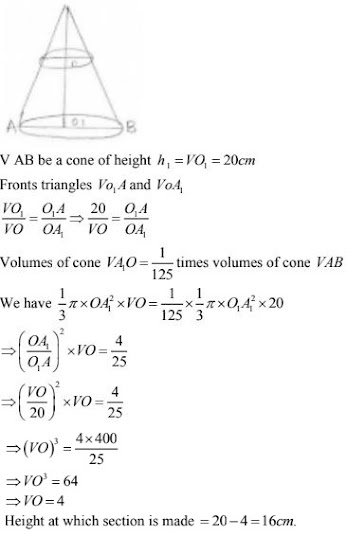7. If the radii of circular ends of a bucket 24cm high are 5cm and 15cm. Find surface area of bucket ?
Solution
Given height of a bucket (R) = 24cm
Radius of circular ends of bucket 5cm and 15cm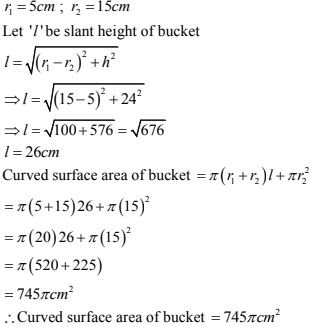8. The radii of circular bases of a frustum of a right circular cone are 12 cm and 3 cm and height is 12 cm. Find the total surface area volume of frustum  ?
Solution
Let slant height of frustum cone be 'I'
Given height of frustum cone 12 cm
Radii of a frustum cone are 12 cm and 23 cm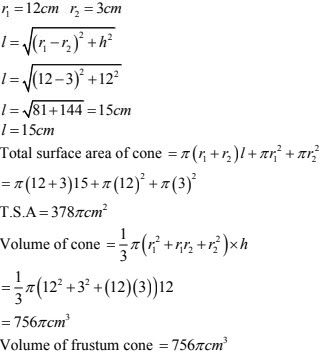9. A tent consists of a frustum of a cone copped by a cone. If radii of ends of frustum be 13 m and 7m the height of frustum be 8m and slant height of thee conical cap bee 12m. Find canvas required for tent ?
Solution
Given height of frustum (h) = 8m
Radii of frustum cone are 13m and 7m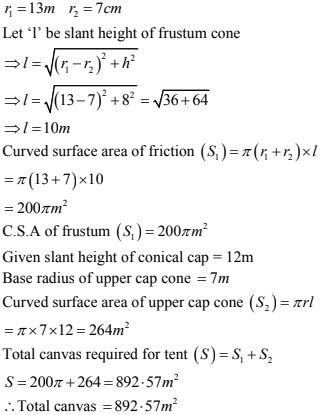10. A reservoir in form of frustum of a right circular contains 44 × 107  liters off water which fills it completely. The radii of bottom and top of reservoir are 50 m and 100 m. Find depth of water and lateral surface area of reservoir ?
Solution
Let depth of frustum cone be h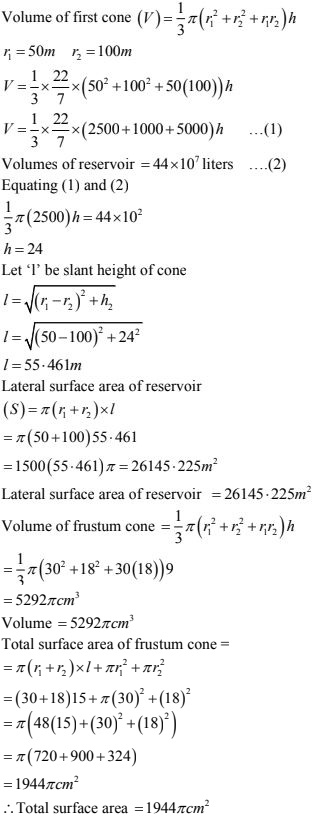11. A metallic right circular cone 20 cm high and whose vertical angle is  90° is cut into two parts at the middle point of its axis by a plane parallel to base. If frustum so obtained bee drawn into a wire of diameter (1/16) cm. find length of the wire ?
Solution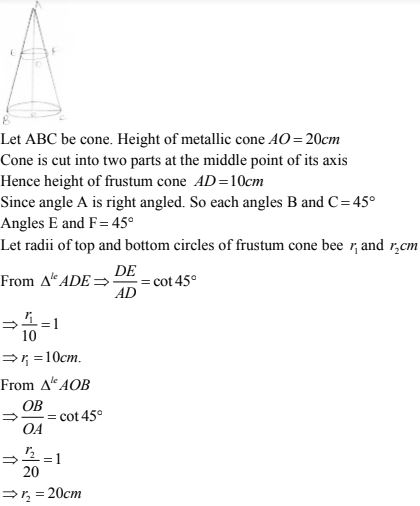12. A bucket is in the form of a frustum of a cone with a capacity of 12308.8 cm3 of water. The radii of the top and bottom circular ends are 20 cm and 12 cm respectively. Find the height of the bucket and the area of the metal sheet used in its making. (Use π = 3.14).
Solution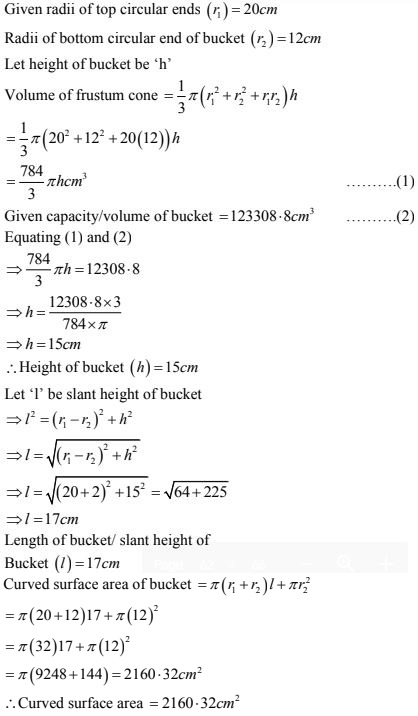13. A bucket made of aluminum sheet is of height 20 cm and its upper and lower ends are of radius 25 cm and 10 cm, find cost of making bucket if the aluminum sheet costs Rs 70 per 100 cm2.
Solution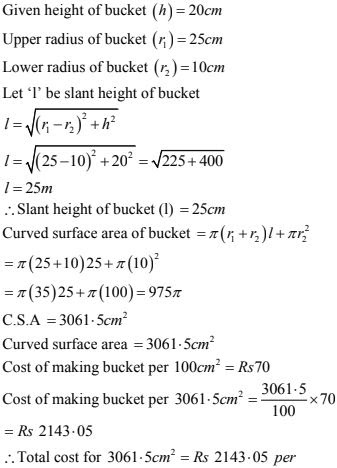14. Radii of circular ends of a solid frustum off a cone are 33 cm and 27 cm and its slant height are 10 cm. Find its total surface area  ?
Solution
Given slant height of frustum cone = 10 cm
Radii of circular ends of frustum cone are 33 cm and 27 cm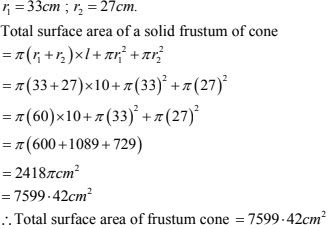15. A bucket made up of a metal sheet is in form of a frustum of cone of height 16cm with diameters of its lower and upper ends as 16 cm and 40 cm. Find the volume of bucket. Also find cost of bucket if the cost of metal sheet used is Rs  20 per 100 cm2   .
Solution
Given height off frustum cone  = 16 cm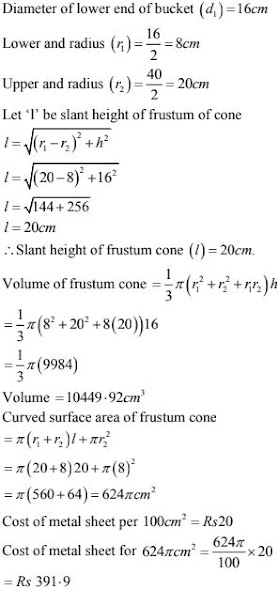∴ Total cost of bucket  = Rs. 391.9

16. A solid is in the shape of a frustum of a cone. The diameter of two circular ends are 60 cm and 36cm and height is 9 cm. Find area of its whole surface and volume ?
Solution
Given height of a frustum cone = 9 cm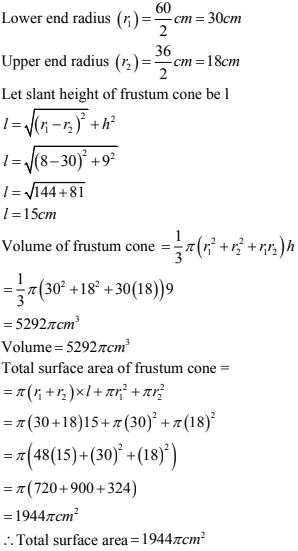17. A milk container is made of metal sheet in the shape of frustum of a cone whose volume is (10459)(3/7) cm3. The radii of its lower and upper circular ends are 8 cm and 20 cm. Find the cost of metal sheet used in making container at rate of Rs 1.4 per cm2?
Solution